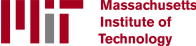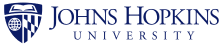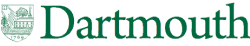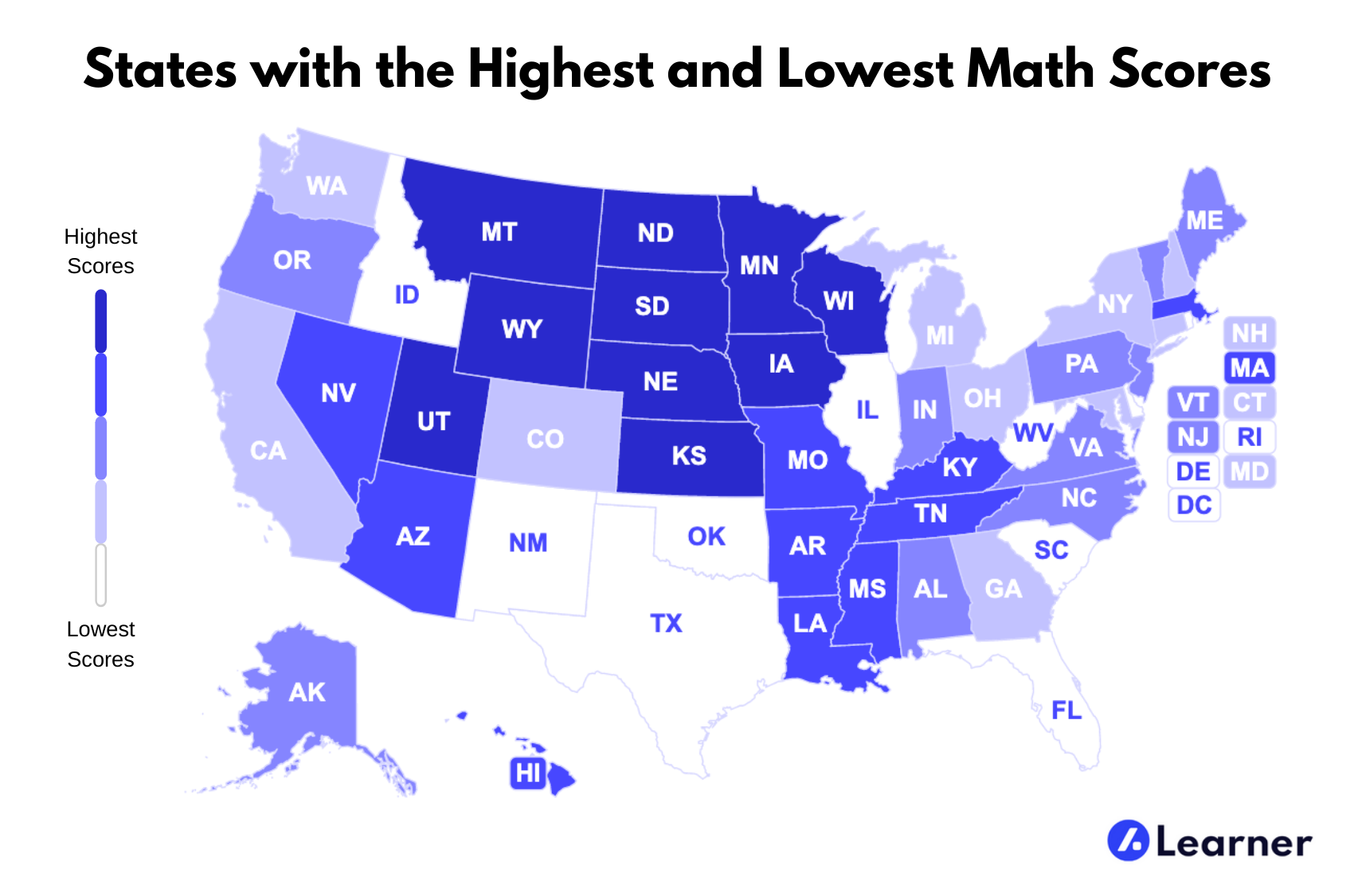# What Is a Complex Conjugate In Mathematics?

In mathematics, every complex number (a two-component number involving a real number added to an imaginary number) has a complex conjugate. This complex conjugate will have the same real part, while the imaginary part will have the same magnitude but the opposite sign. A complex number can be represented by the expression a + bi, and its complex conjugate can be represented by a - bi, where a and b are real numbers and i is an imaginary number (specifically, the square root of negative 1).

Despite having imaginary components, complex conjugates are used to describe physical realities. The use of complex conjugates works despite the presence of imaginary components, because when the two components are multiplied together, the result is a real number. In terms of their mathematical applications, complex conjugates are used in the rationalization of complex numbers. Math tutoring is an excellent way to help students understand complex conjugates as they’re also necessary for finding the amplitude of the polar form of a complex number.## Real Number Component

Every complex number possesses a real number component added to an imaginary component. In the expression a + bi, a is the real number component.

## Imaginary Number Component

An imaginary number is defined as any number that when squared results in a real negative number. This may be restated in other terms for simplification. An imaginary number is any real number multiplied by the square root of negative one (-1). In this form, a complex conjugate is a pair of numbers that can be written y = a+bi and ӯ = a–bi, where “i” is the square root of -1. To distinguish the two y-values, one is generally written with a bar over the letter, ӯ, although occasionally an asterisk is used.

Though their magnitude is equal, the sign of one of the imaginary components in the pair of complex conjugate numbers is opposite to the sign of the other.

## Where Did Complex Numbers Come From?

The basic idea that a complex number exists in a number system containing real numbers and imaginary numbers originated with the mathematician Gerolamo Cardano in 1545, though many other mathematicians have also contributed to the concept of complex numbers, such as Casper Wessel in 1799.

The earliest reference to the square root of a negative number (i.e., an imaginary number) is thought to appear in the work of the ancient Greek mathematician Hero of Alexandria. Regardless of the lengthy history of complex numbers, there are many ways in which they’re applied today, including in multiplication.

## Multiplication of Two Complex Conjugates

To demonstrate that the multiplication of two complex conjugate numbers produces a real result, consider the example y = 7+2i and ӯ = 7–2i. Multiplying them together gives yӯ = 49+14i–14i–4i2 = 49+4 = 53. Such a real result from complex conjugate multiplication is important, particularly when considering systems at the atomic and subatomic levels. Frequently, the mathematical expressions for minuscule physical systems include an imaginary component. This is especially important in quantum mechanics, which is the non-classical physics of the very small.

## Equations in Quantum Mechanics

In quantum mechanics, the characteristics of a physical system consisting of a particle are described by a wave equation. These equations reveal everything that can be learned about the particle in its system. Frequently, wave equations feature an imaginary component. Multiplying the equation by its complex conjugate results in a physically interpretable “probability density.” The characteristics of the particle may be determined by mathematically manipulating this probability density.

## ‍”Born Probability”

As an example, the use of probability density is important in the discrete spectral emission of radiation from atoms. This application of probability density is called “Born probability,” after the German physicist Max Born. The closely-related statistical interpretation that the measurement of a quantum system will give certain specific results is called the Born rule. Max Born received the 1954 Nobel Prize in Physics for his work in this area. Unfortunately, attempts to derive the Born rule from other mathematical derivations have been met with mixed results.

## Which Professions Use Complex Numbers?

There are a few obvious professionals who use complex numbers, including scientists, engineers, and, of course, mathematicians. Physicists, quantum physicists, and electrical engineers also use complex numbers on a regular basis. And there are other, less obvious professions that rely on complex numbers. Interestingly, sales analysts and economists regularly use complex numbers in their line of work.

Complex numbers can also be used in signal processing to find certain notes with audio-editing software. They are involved in AC circuit analysis, as well. Complex numbers are frequently utilized in calculations of current, voltage, and resistance in AC circuits. This data can then be used to calculate the differences between two AC power supplies over time. And as mentioned above, complex numbers play an important role in quantum mechanics, the branch of physics that studies the motions and interactions between subatomic particles.

These are just a few examples of how complex numbers (and complex conjugates) are used for practical, real-world purposes. It’s important for students to have a firm grasp of complex numbers and related topics because they are essential to a wide variety of professions. Math problems involving complex numbers may also appear on certain college entrance exams.

## Do High School Students Use Complex Conjugation?

Whether a high school student learns complex conjugation depends on which mathematics classes are taught in a particular school. Classes often vary from state to state, so it’s hard to say exactly what will be taught in high school classrooms. Often, complex conjugates are taught in Algebra 1 or Algebra 2. However, complex conjugates are not just restricted to Algebra courses, as instructors could discuss real and imaginary numbers in varying contexts.

Typically, Algebra I is taught to ninth grade students or eighth grade honor students. Some students may take Algebra 2 during their junior year of high school.

## Are Complex Numbers on the SAT Test?

Most of the SAT Math section is focused on numbers, operations, algebra, functions, geometry, measurement, data analysis, statistics, and probability. That being said, there is a chance that the concept of complex numbers could appear on the SAT in one or two questions. In order to be well prepared, it’s a good idea to have a firm grasp of complex numbers and complex conjugates before taking the SAT.

There are many components that work together to yield a correct answer in mathematics, so it’s important for students to know how to do certain procedures and how to use certain techniques across various mathematical subjects. It can be difficult to determine what an SAT question is asking if a student does not have an in-depth knowledge of several branches of mathematics.One of the best ways to prepare for college entrance exams and ensure success is to work with a tutor. A tutor can tailor their instruction to a student’s specific needs. Contrary to what some might think, tutors can greatly benefit  all students, regardless of whether they’re struggling with certain concepts or whether they excel in class and need something more challenging.

## How Learner Math Tutors Can Help

While math may come easily to some, there are many mathematical topics that can be confusing for high school and college students. Mathematics is a complex, multifaceted subject. Certain concepts may seem overwhelming, but an experienced math tutor can help. A tutor can assist students by providing individualized help and instruction – depending on which tutoring service the parent or student chooses. Some companies only offer generic lessons based on the state curriculum and standardized tests, while others provide a far more individualized experience. The best tutoring services will first ascertain the strengths and weaknesses of the student and then base tutoring sessions on their learning needs and current academic performance.

Learner’s tutoring model is based on personalized lessons that are intended to help students grasp the concepts they find most difficult, yet need to master for their classes or exams. The process of connecting with a Learner tutor is very simple. First, parents answer questions about their child’s academic background and unique needs. Then, they schedule a call with an Academic Coach to identify their child’s learning goals and objectives. Lastly, Learner will custom match each child with the right tutor. The first session with Learner is always free, so it’s a great opportunity to try out tutoring and see if it’s a good fit.

Learner understands that every student has their own learning style, unique way of thinking, and academic aspirations. Working with a highly experienced Learner tutor is a great way to give specific, direct feedback to students on their performance. Tutoring is also an excellent way to encourage students and build their confidence as they tackle new and unfamiliar topics, such as complex conjugates.

Keep in mind that tutors aren’t just for students who need extra academic help. Tutoring is a great resource for students who are excelling academically, too. Tutors can encourage these students to think creatively, give them additional practice, and stretch and challenge them beyond the basics of the school curriculum.

Looking for private one-on-one math tutoring? Speak with our academic advisor to get custom matched with your online math tutor today!

Complex conjugates can be a confusing topic. Here are some common questions and answers about complex conjugates and related topics.

### What Is the Square Root of a Complex Number?

The square root of a complex number can be determined by using a formula. The square root of a natural number comes in pairs, and the square root of a complex number uses x and y to represent real numbers.

### What Is a Complex Plane in Math?

A complex plane is the plane formed by complex numbers. The complex plane has an x-axis, which is called the real axis and is formed by real numbers. The complex plane also has a y-axis, which is called the imaginary axis because it is formed by imaginary numbers.

### What Are Real Coefficients?

A coefficient is any modifying value associated with a variable by multiplication. A real number is any non-imaginary number.

### What Is a Notation in Math?

In math, notations are symbols used to represent mathematical objects and ideas. Mathematical notations are essential to the physical sciences, engineering, and economics. Common notations include symbols representing approximation, greater than, less than, inequality, addition, subtraction, multiplication, and division.

### What Is the Product of Two Complex Numbers?

When two imaginary numbers are multiplied together, the product is a real number.

### Can Complex Numbers Be Used in Fractions?

If you have a fraction with a complex number in the denominator, you can multiply both the numerator and the denominator by the complex conjugate of the denominator. This simplifies the fraction rather than changing the value of the fraction.

### Can Complex Numbers Be Negative?

A complex number can be the negative of another complex number, but it cannot itself be negative.

## Latest Articles### States That Spend the Most on Education

Investing in the future of our children via their education can help them build the lives they want. But education comes at a price—and a high one, at that. And some states invest more in their students than others. Find out which states have the highest spending per student!

Mathematics### States with the Highest and Lowest Math Scores

At Learner, we understand both the difficulty and the necessity of math as a subject and wanted to know what math looks like in the United States. We took a look at the average SAT math scores throughout the nation to find which states had the highest and the lowest. Find out how your state stacks up against the rest!

Mathematics### How Can I Score Well on the GED® Math Test?

Passing the General Education Development® (GED®) test gives a person the equivalent of a high school diploma. The math test is one of five sections of the exam. To score well on the GED® math test, you need a sound understanding of the concepts tested in each of the four content areas covered in this section.

Mathematics